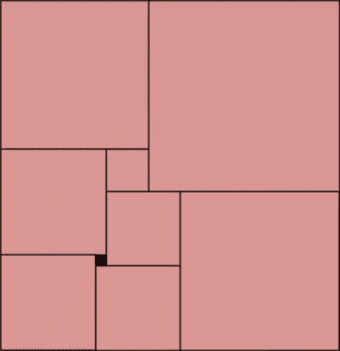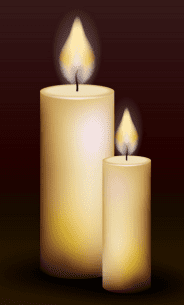## Poll

 I love math!8 votes (47.05%) Math is great.6 votes (35.29%) My religion is mathology.3 votes (17.64%) Women didn't speak to me until I was 30.2 votes (11.76%) Total eclipse reminder -- 04/08/20246 votes (35.29%) I steal cutlery from restaurants.2 votes (11.76%) I should just say what's on my mind.3 votes (17.64%) Who makes up these awful names for pandas?2 votes (11.76%) I like to touch my face.8 votes (47.05%) Pork chops and apple sauce.6 votes (35.29%)

17 members have voted

charliepatrickJoined: Jun 17, 2011
• Posts: 2111
Thanks for this post from:July 29th, 2020 at 10:32:08 AM permalink
If you call the squares starting at the top, A B, C D, E F, G H. Then if you start at the square NE of the small square (E). Then work clockwise.
(i) H=E+4, consider its top edge which meet "4" and "E".
(ii) G=H+4=E+8, consider its Eastern edge which meet "4" and "H".
(iii) Similarly C=G+4=E+12.
(iv) The height of C and G is the same as D, E and H. (E+12)+(E+8)=D+E+(E+4). So D=16.
(v) A= C+D = (E+12)+16 = E+28.
(vi) B= A+C = (E+28)+16 = E+44.
(vii) F=E+H = 2E+4.
(viii) The width of the top row A+B = the width of the bottom row F+G+H = (E+28)+(E+44) = (E+8)+(E+4)+(2E+4); 2E+72=4E+16; 72-16=2E; E=28.

Width = A+B = 2E+72= 128
Height = A+C+G = (E+28)+(E+12)+(E+8) - 3E+48 = 132.

So area = 128*132 = 16896.
ChesterDogJoined: Jul 26, 2010
• Posts: 875
Thanks for this post from:July 29th, 2020 at 10:37:56 AM permalink
Quote: Gialmere...

Since this is an easy math puzzle, I did it an easy way.

I copied the picture using Microsoft's Snipping Tool and pasted into a new "drawing canvas" in a Word document.

I found that a square 0.1 inch on a side covered the picture of the little square. The other eight squares were 0.4, 0.7, 0.8, 0.9, 1.0, 1.4, 1.5, and 1.8 inches on a side. (Also, I checked that sums of adjacent groups of squares were consistent with these measurements.) My rectangle was 1.4 + 1.8 = 3.2 inches wide and 1.8 + 1.5 = 3.3 inches tall.

Therefore, the original rectangle is 40(3.2) = 128 cm wide and 40(3.3) = 132 cm tall for an area of (128)(132) = 16,896 cm2.
ThatDonGuyJoined: Jun 22, 2011
• Posts: 4278
July 29th, 2020 at 2:01:50 PM permalink
A Mersenne Prime is a prime number that is one less than a power of 2.
For example, 127 is a Mersenne prime, as 127 = 27 - 1, and 7 is prime.
Note that the power of 2 must also be prime, as 2 to the power of (a nonprime number > 1) - 1 cannot be prime.

In March of 2011, someone claimed to have found two Mersenne primes (prime numbers that are one less than powers of 2); one ended in the digits 785894583, and the other in 943626843.
Prove that neither of these numbers could possibly be a Mersenne prime.
charliepatrickJoined: Jun 17, 2011
• Posts: 2111
July 29th, 2020 at 2:09:35 PM permalink
Quote: ThatDonGuy

...one ended in the digits 785894583, and the other in 943626843.
Prove that neither of these numbers could possibly be a Mersenne prime.

The last digits in powers of 2 go in a cycle of 4.

2^1 = 2
2^2 = 4
2^3 = 8
2^4 =16
2^5 =32
2^6 =64

Thus 2^(4n+1)=10x+2.
Thus 2^(4n+2)=10x+4.
Thus 2^(4n+3)=10x+8.
Thus 2^(4n+4)=10x+6.

Thus an odd power of 2 ends in either a 2 or 8.

So a mersenne prime ends in 1 or 7; i.e. not a 3,
ThatDonGuyJoined: Jun 22, 2011
• Posts: 4278
Thanks for this post from:July 29th, 2020 at 2:46:09 PM permalink
Quote: charliepatrick

The last digits in powers of 2 go in a cycle of 4.

2^1 = 2
2^2 = 4
2^3 = 8
2^4 =16
2^5 =32
2^6 =64

Thus 2^(4n+1)=10x+2.
Thus 2^(4n+2)=10x+4.
Thus 2^(4n+3)=10x+8.
Thus 2^(4n+4)=10x+6.

Thus an odd power of 2 ends in either a 2 or 8.

So a mersenne prime ends in 1 or 7; i.e. not a 3,GialmereJoined: Nov 26, 2018
• Posts: 1599
July 29th, 2020 at 4:17:47 PM permalink
Quote: ThatDonGuy

Quote: Gialmere

The rectangle above is divided into 9 perfect squares.

The smallest square (colored black) has a side length of 4cm.

What is the area of the rectangle?

Label the eight squares as follows:
A - upper left
B - upper right
C - left edge, below A
D - touching A, B, C
E - below D
F - bottom left
G - bottom center
H - bottom right

G = E + 4
F = G + 4 = E + 8
C = F + 4 = E + 12
C + 4 = D + E -> E + 16 = D + E -> D = 16

A = C + D = E + 12 + 16 = E + 28

B = A + D = E + 44

B + D = E + H -> E + 44 + 16 = E + H -> H = 60

H = E + G = 2E + 4 -> E = 28

The eight squares A, B, C, D, E, F, G, H have side lengths 56, 72, 40, 16, 28, 36, 32, and 60, respectively

The width of the rectangle = A + B = 128
Check: it is also F + G + H = 128
The height of the rectangle = A + C + F = 132
Check: it is also B + H = 132
The area = 128 x 132 = 16,896

Quote: charliepatrick

If you call the squares starting at the top, A B, C D, E F, G H. Then if you start at the square NE of the small square (E). Then work clockwise.
(i) H=E+4, consider its top edge which meet "4" and "E".
(ii) G=H+4=E+8, consider its Eastern edge which meet "4" and "H".
(iii) Similarly C=G+4=E+12.
(iv) The height of C and G is the same as D, E and H. (E+12)+(E+8)=D+E+(E+4). So D=16.
(v) A= C+D = (E+12)+16 = E+28.
(vi) B= A+C = (E+28)+16 = E+44.
(vii) F=E+H = 2E+4.
(viii) The width of the top row A+B = the width of the bottom row F+G+H = (E+28)+(E+44) = (E+8)+(E+4)+(2E+4); 2E+72=4E+16; 72-16=2E; E=28.

Width = A+B = 2E+72= 128
Height = A+C+G = (E+28)+(E+12)+(E+8) - 3E+48 = 132.

So area = 128*132 = 16896.

Quote: ChesterDog

Since this is an easy math puzzle, I did it an easy way.

I copied the picture using Microsoft's Snipping Tool and pasted into a new "drawing canvas" in a Word document.

I found that a square 0.1 inch on a side covered the picture of the little square. The other eight squares were 0.4, 0.7, 0.8, 0.9, 1.0, 1.4, 1.5, and 1.8 inches on a side. (Also, I checked that sums of adjacent groups of squares were consistent with these measurements.) My rectangle was 1.4 + 1.8 = 3.2 inches wide and 1.8 + 1.5 = 3.3 inches tall.

Therefore, the original rectangle is 40(3.2) = 128 cm wide and 40(3.3) = 132 cm tall for an area of (128)(132) = 16,896 cm2.

Correct! Including a Kobayashi Maru solve.
--------------------------------

What do you get when you cross two squares with an ogre?

A Shrektangle.
Have you tried 22 tonight? I said 22.
GialmereJoined: Nov 26, 2018
• Posts: 1599
July 30th, 2020 at 8:00:02 AM permalinkA college student was studying for exams late at night when a power blackout occurred.

He lit two uniform candles of equal length but one thicker than the other. The thick candle could burn for four hours and the thin one an hour less. When he finally went to sleep, the thick candle was twice as long as the thin one.

How long did the student study by candle light?
Have you tried 22 tonight? I said 22.
charliepatrickJoined: Jun 17, 2011
• Posts: 2111
Thanks for this post from:July 30th, 2020 at 8:54:19 AM permalink
Let's assume the candles were originally 12" tall. So the large one (L) burns at 3" per hour, and the small one (S) at 4" per hour.

After 2 hours L has lost 6" so has 6" left; S has lost 8" so has 4" left. After 3 hours L has lost 9" so has 3" left, S has now just burnt out.

So let t be the time before 3 hours. The height of the large one is L=3+3t, the height of the small one is S=4t.
At the time of the solution L=2S.
So 8t=3+3t; 5t=3, t=3/5.

So after 2 2/5 hour...
...the large candle is 12-(2.4*3) = 12-7.2 = 4.8" tall
...the small candle is 12-(2.4*4) = 12-9.6 = 2.4" tall.

So the time is 2 hours 24 minutes.
WizardJoined: Oct 14, 2009
• Posts: 21923
Thanks for this post from:July 30th, 2020 at 10:59:41 AM permalink

I see I agree with Charlie, but I want to get credit anyway...

Let:
a = Time remaining on thick candle
b = Time remaining on thin candle
t = Time candle burned.

a = 4-t
b = 3-t

The height of each candle will be proportional to it's remaining time to it's beginning time.

Ratio of height left of a = (4-t)/4
Ratio of height left of b = (3-t)/3

Let's say each candle starts at 12". At some time x, the thick one will be twice as tall. In other words,

12*((4-t)/4) = 2*12*((3-t)/3)
3 * (4-t) = 8 * (3-t)
12 - 3t = 24 - 8t
5t = 12
t = 2.4

So, the candles have burned for 2.4 hours.

Good puzzle.
It's not whether you win or lose; it's whether or not you had a good bet.
USpapergamesJoined: Jun 23, 2020